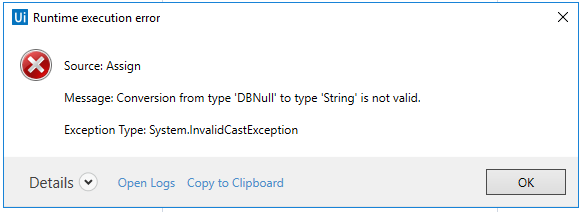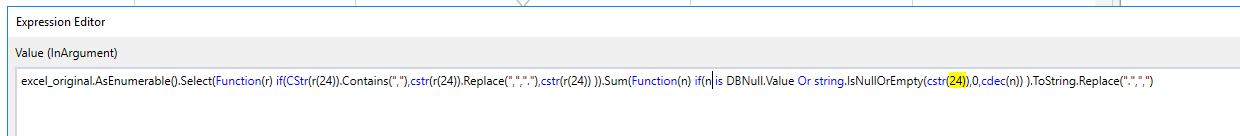# How to total up all values of a specific column to a single sum

I would like to total up all values/cells of a specific column and store the sum in a variable to keep on working with it.
So far I implemented this:
String_var = excel_original.AsEnumerable.Sum(Function(x)Convert.ToDecimal(x(10))).ToString

The problem is that this code does not consider the commas which are the decimal separators.
So in the case below

1,5
2,5
3
2,5
3,5

… the result should actually be 13. But it’s 130 since the comma is not considered.
Can someone please help?Convert.ToDecimal(value, System.Globalization.CultureInfo(“fr-FR”))

@bcorrea
Like this…

String_var = excel_original.AsEnumerable.Sum(Function(x)Convert.ToDecimal(value, System.Globalization.CultureInfo(“fr-FR”)).ToString

???

if you have a format that is different from the region format that your system is running on, than you need to specify the right one…

Hello @MarcJo

You can easily do that using LinQ

Use this Code

`DT.AsEnumerable().Select(Function(r) if(CStr(r("A")).Contains(","),cstr(r("A")).Replace(",","."),cstr(r("A")) )).Sum(Function(n) cdec(n) )`

This code will replace the Commas ( , ) with Dots (.) and then sum all the value check the code and let me know about it

1 Like

Hi @vickydas,
The point is that the values’ format has to be kept for following processing.
So is there an opportunity to total it up while keeping the comma?

Hello @MarcJo

By this you mean to keep the comma in the desired output right ? because the input data is not affected by the code…

For this output after getting the sum you can simply just replace the Dot with a Comma
just like this

`DT.AsEnumerable().Select(Function(r) if(CStr(r("A")).Contains(","),cstr(r("A")).Replace(",","."),cstr(r("A")) )).Sum(Function(n) cdec(n) ).ToString.Replace(".",",")`

1 Like

@vickydas …sounds good.
And the “A” stands for the column?
So I need to replace it with the desired column I actually want to be totaled up?

@MarcJo

Yes

Yes “A” here is the column name

@vickydas… it’s working!Thank you so much, you’re my saviorHi @vickydas,
sorry for disturbing you again.
But I tried to adopt your code for a similar process and this was not working - see screenshot below:

I guess it’s only a minimal adjustment but I currently don’t know on which position in the code.
Can you please help me once again?Hello @MarcJo

This error occured because you have blank values in your data SO it cannot blank value to Decimal

`DT.AsEnumerable().Select(Function(r) if(CStr(r("A")).Contains(","),cstr(r("A")).Replace(",","."),cstr(r("A")) )).Sum(Function(n) if(string.IsNullOrEmpty(cstr(n)),0,cdec(n)) ).ToString.Replace(".",",")`

This code will consider a blank value as 0 and will give you desired output…

Also you can learn basic methods of LinQ from the link below
LINQ samples in LINQ to Objects

@vickydas thanks a lot for your help@vickydas,
sorry for asking you again but I got another error message I don’t know how to get rid of:Could you help one last time please?

change this part `string.IsNullOrEmpty(cstr(n))` to this `n is DBNull.Value Or string.IsNullOrEmpty(cstr(n))`

@bcorrea I changed the code accordingly but the error message was the sameAny other idea?

ok, then is because the column that is bringing null values is the r(24), you will need to add to that if condition the same as you did for for it…

@bcorrea, @vickydas
After changing it accordingly I received the same error message…excel_original.AsEnumerable().Select(Function(r) if(CStr(r(24)).Contains(“,”),cstr(r(24)).Replace(“,”,“.”),cstr(r(24)) )).Sum(Function(n) if(n is DBNull.Value Or string.IsNullOrEmpty(cstr(24)),0,cdec(n)) ).ToString.Replace(“.”,“,”)

in here: excel_original.AsEnumerable().Select(Function( r ) if(CStr(r(24)).

@bcorrea would you mind sending me the whole code?
For now I am not really sure which changes still need to be done